# Kerala Syllabus 9th Standard Maths Solutions Chapter 6 Parallel Lines

## Kerala State Syllabus 9th Standard Maths Solutions Chapter 6 Parallel Lines

### Kerala Syllabus 9th Standard Maths Parallel Lines Text Book Questions and Answers

Textbook Page No. 88

Parallel Lines Class 9 Kerala Syllabus Question 1.
Draw an 8 cm long line and divide it in the ratio 2 : 3.
Draw AB whose length is 8 cm. Draw AC with length 5 cm. Join BC. Let the point D divides AC in the ratio 2 : 3. Draw a line through D parallel to BC. This line divides AB in the ratio 2 : 3.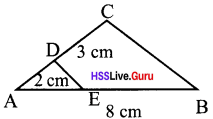Kerala Syllabus 9th Standard Maths Chapter 6 Question 2.
Draw a rectangle of perimeter 15 cm and sides in the ratio 3 : 4
Perimeter is 15 cm.
So length + breadth = 7.5 cm
Draw AB, a line segment of length 7.5 cm.
Draw AC from A, 4 + 3 = 7cm long.
Mark D on this line such that AD = 4 cm. Draw BC.
Draw a line parallel to BC and passing through D, which meets AB at E. Draw rectangle AEGF whose length as AE and breadth as EB.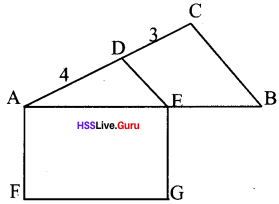Class 9 Maths Parallel Lines Kerala Syllabus Question 3.
Draw rectangles specified below each of perimeter 10 cm.
i. Equilateral triangle.
ii. Sides in the ratio 3 : 4 : 5
iii. Sides in the ratio 2 : 3 : 4
i. Draw a line AB of length 10 cm.
Draw AC with length 9 cm. Join BC.
AD = DE = EC = 3
AF : FG : GB = 3 : 3 : 3 = 1 : 1 : 1
Divide it in the ratio 1 : 1 : 1 and draw a triangle.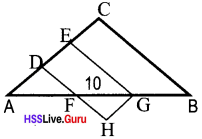Draw ΔFHG with FG as its side.

ii. Draw a line segment AB of length 10 cm. Draw AC = 12 cm, join BC. Divide it in the ratio 3 : 4 : 5.
AD : DE : EC = 3 : 4 : 5
AF : FG : GB = 3 : 4 : 5
Draw ΔGHB with sides AF, FG and GB.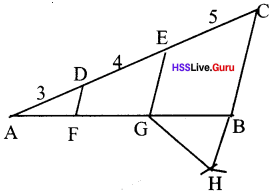iii. Draw a line segment AB of length 10 cm. Draw AC with length 9 cm, join BC. Divide it in the ratio 2 : 3 : 4.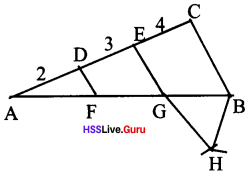AD : DE : EC = 2 : 3 : 4
AF : FG : GB = 2 : 3 : 4
Draw ΔGHB with sides AF, FG and GB.

Parallel Lines 9th Standard Kerala Syllabus Question 4.
In the picture below, the diagonals of the trapezium ABCD intersect at P. Prove that PA × PD = PB × PC.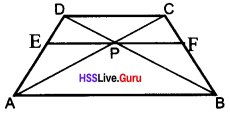Sides AB, CD are parallel.
Draw EF parallel to AB and passing through P.
Now lines AB, EF and DC are parallel.
These lines cut the lines AC and BD in the same ratio.
So, PA : PC = PB : PD
i.e., $$\frac {PA}{PC} = \frac {PB}{PD}$$
From this by cross multiplication we get PA × PD = PC × PB

Textbook Page No. 93

Parallel Lines Class 9 Kerala Syllabus Question 1.
In the picture, the perpendicular is drawn from the midpoint of the hypotenuse of a right triangle to the base. Calculate the length of the third side of the large right triangle and the lengths of all three sides of small right triangle.AC = 10 cm
∴ AM = 5 cm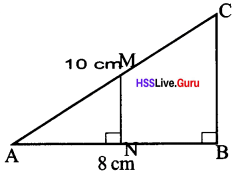MN, CB are perpendicular to AB
MN, CB are parallel lines.
∴ MN divides AB and AC in the same ratio.
∴ AN = BN = 4cm
BC = $$\sqrt{10^{2} – 8^{2}} = \sqrt {100 – 64} = \sqrt {36}$$ = 6 cm
M is the midpoint of the AC. N is the midpoint of AB.
The length of the line joining the midpoints of two sides of a triangle is half the length of the third side. So MN is half of BC.
∴ MN = 3cm

Parallel Lines Chapter Class 9 Kerala Syllabus Question 2.
Draw a right triangle and the perpendicular from the midpoint of the hypotenuse to the base.i. Prove that this perpendicular is half the perpendicular side of the large triangle.
ii. Prove that perpendicular bisects the bottom side of the larger triangle.
iii. Prove that in the large triangle the distances from the mid point of the hypotenuse to all the vertices are equal.
iv. Prove that the circumcentre of a right triangle is the mid point of its hypotenuse.
MN is perpendicular to AB.
MN and CB are parallel lines. MN divides AC and AB in the same ratio.i. AM = $$\frac{1}{2}$$AC, AN = $$\frac{1}{2}$$AB
∴ MN = $$\frac{1}{2}$$CB

ii. We get two right triangles of same base and perpendicular. So their hypotenuses are also equal.
∴ MA = MC = MB
AN = NB

iii. ∠ANM = ∠BNM = 90°
MN = MN
ΔANM ≅ ΔBNM
AM = MB …….(1)
M is the midpoint of AC
AM = MC …….(2)
(1) = (2) ⇒ AM = MB = MC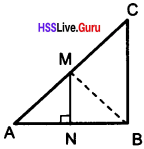iv. The points A, B and C are at same distance from M. So a circle can be drawn with M as centre and pass through there three points. So this circle is the circum circle of ΔABC.

9th Standard Maths Notes Kerala Syllabus Question 3.
In the parallelogram ABCD, the line drawn through a point P on AB, parallel to BC, meets AC at Q. The line through Q, parallel to AB meets AD at R.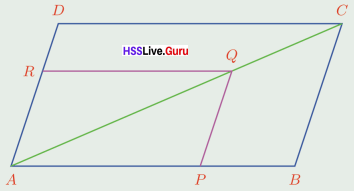Prove that $$\frac {AP}{PB} = \frac {AR}{RD}$$
In ΔABC, RQ the parallel line of BC, divides AB and BC in the same ratio When we consider the relation
$$\frac {AR}{RD} = \frac {AQ}{QC}, \frac {AP}{PB} = \frac {AQ}{AC} = \frac {AR}{RD}$$$$\frac {AR}{RD} = \frac {AQ}{QC}$$
$$\frac {AP}{PB} = \frac {AQ}{AC} = \frac {AR}{RD}$$
∴ $$\frac {AP}{PB} = \frac {AR}{RD}$$

Hsslive Guru 9th Maths Kerala Syllabus Question 4.
In the picture below, two vertices of a parallelogram are joined to the mid points of two sides. Prove that these lines divide the diagonal in the picture into three equal parts.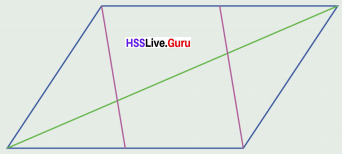In the parallelogram ABCD, AB = CD and AB parallel CD.
P is the mid point of AB and Q is the midpoint of DC.
Since P and Q are the midpoints of AB and CD respectively.
PB = ½AB
DQ = ½CD = ½AB
∴ PB = DQ
i.e., PB = DQ and PB parallel DQ.
∴ PBQD is a parallelogram.
Since the parallel lines PD and BQ cut AB into equal parts, they cut AY also into equal parts.
AX = XY
The parallel lines PD and BQ cut CD into equal parts. So they cut CX also into equal parts.
YC = XY
∴ AX = XY = YC

9th Standard Maths Kerala Syllabus Question 5.
Prove that the quadrilateral formed by joining the mid points of a quadrilateral is a parallelogram. What if the original quadrilateral is a rectangle? What if it is a rhombus?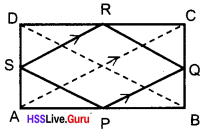P, Q, R and S are the midpoints of the sides of the quadrilateral ABCD. Draw the diagonals AC and BD.
The length of the line joining the mid-points of two sides of a trianglle is half the length of the third side.
In ΔABC, PQ = ½AC
∴ PQ = SR
In ΔABD, PS = ½BD
In ΔBCD, QR = ½BD
∴ PS = QR
In quadrilateral PQRS, both pairs of opposite sides are equal. So it is a parallelogram.
PQ = ½AC, RS = ½AC
∴ RS = PQ = ½AC
PS = ½BD, QR = ½BD
∴ PS = QR = ½BD = ½AC
∴ PQ = RS = PS = QR
All sides of the quadrilateral PQRS are equal. So it is a rhombus.
Since ABCD is a rhombus. AC perpendicular to BD.
PQ and SP are perpendicular, i.e., ∠SPQ = 90°
One angle of the parallelogram PQRS is 90° and therefore it is a rectangle.

### Kerala Syllabus 9th Standard Maths Parallel Lines Exam Oriented Text Book Questions and Answers

Class 9 Maths Chapter 6 Kerala Syllabus Question 1.
Draw ΔABC with AB = 5cm, BC = 4cm, AC = 6cm, ΔABC. In BC mark P such that BP = 1cm, PC = 3cm. What is the relation between the areas of ΔABP and ΔAPC?
Area of ΔAPC is 3 times the area of ΔABP.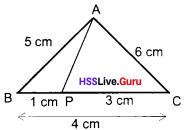If two triangles have the common vertex and their bases are along the same straight line, the ratio between their areas is equal to the ratio between the lengths of their bases.
⇒ $$\frac{Area of ΔABP}{Area of ΔAPC} = \frac{BP}{PC} = \frac{1}{3}$$

Chapter 6 Maths Class 9 Kerala Syllabus Question 2.
In ΔABC, the line parallel to BC meet AB and AC at D and E respectively. AE = 4.5cm, $$\frac{AD}{DB} = \frac{2}{5}$$ = then find EC.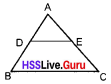$$\frac{AD}{DB} = \frac{AE}{EC}$$
$$\frac{2}{5} = \frac{4.5}{EC}$$ = EC = $$\frac{4.5 \times 5}{2} = \frac{22.5}{2}$$
= 11.25 cm

Hsslive Guru Maths 9th Kerala Syllabus Question 3.
In ΔPQR, PQ = 9cm, QR = 14 cm, PR = 12 cm The bisector of ∠PRS meets QR in S. Find QS and RS.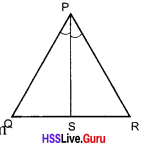$$\frac{PQ}{PR} = \frac{QS}{SR}$$;
$$\frac{18}{24} = \frac{6}{RS}$$
= RS = $$\frac{24 \times 6}{18}$$ = 8 cm

Hsslive Guru 9 Maths Kerala Syllabus Question 4.
In ΔABC, the point P is on AB such that the length of AP is double the length of PB. The line through P parallel to BC meets AC at Q and the length of AQ is 1 cm more than that of QC, what is the length of AC?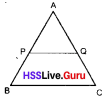AP = 2 × PB
AP : PB = 2 : 1
$$\frac{AP}{PB} = \frac{AQ}{QC} = \frac{2}{1}$$ ⇒
AQ = 2QC
Consider QC = x cm
x + 1 = 2x ⇒ x = 1 ⇒ QC = 1
AQ = 2 × 1 = 2
AC = AQ + QC = 2 + 1 = 3 cm

Hss Live Guru Maths Kerala Syllabus Question 5.
In ΔABC, a line parallel to BC cuts a B and AC at X and Y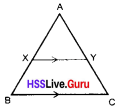(a) If AB = 3.6cm, AC = 2.4cm, AX = 2.1 cm, what is the length of AY?
(b) If AB = 2cm, AC = 1.5cm, AY = 0.9cm
what is the length of BX?
a. $$\frac{AB}{AX} = \frac{AC}{AY} ⇒ \frac{3.6}{2.1} = \frac{2.4}{AY}$$;
⇒ 3.6 × AY = 2.4 × 2.1 ⇒ AY = 1.4 cm

b. Let length of AX = xcm
$$\frac{AX}{AB} = \frac{AY}{AC} ⇒ \frac{x}{2} = \frac{0.9}{1.5}$$;
1.5 × x = 1.8 ⇒ x = 1.2;
BX = AB – AX; BX = 2 – 1.2 = 0.8 cm

Hss Live Guru Maths 9 Kerala Syllabus Question 6.
In the figure, ABCD is a trapezium, and AB || CD. P is the midpoint of AD. If PQ || AB, then prove that Q is the midpoint of BC.Given PQ || AB,
therefore
PQ || DC also. Line segments AD and BC are dividing 3 parallel lines EC, PQ and AB. Given P is the midpoint of AD
⇒ DP = PA ∴ $$\frac{DP}{PA}$$ = 1, ∴ $$\frac{CQ}{QB}$$ = 1
∴ CQ = QB ∴ Q is the midpoint of BC.

Ch 6 Maths Class 9 Kerala Syllabus Question 7.
In the figure AB || EH || DC, Answer the following questions given below.a. If DE = 3cm, EA = 4cm and DG = 5cm, find GB?
b. If BH = 6cm,CB = 16cm and DB = 20cm, find DG?
c. If DA = 36cm, EA = 22cm and DG = 15cm find DB?
d. If DE = 20cm, EA = 25cm and HB = 22cm, find CH?
a. In ΔDAB, AB || EG
∴ $$\frac{DE}{EA} = \frac{DG}{GB}; \frac{3}{4} = \frac{5}{GB} \Rightarrow GB = \frac{20}{3} = 6 \frac{2}{3}$$cm

b. In ΔBDC, DC || GH
∴ $$\frac{BH}{BC} = \frac{BG}{BD}; \frac{6}{16} = \frac{BG}{20}$$;
BG = $$\frac{120}{16} = 7\frac{1}{2}$$cm
DG = BD – BG = 20 – $$7\frac{1}{2}$$ = $$12\frac{1}{2}$$cm

c. In ΔABD, AB || EG
$$\frac{DA}{EA} = \frac{DB}{BG}; \frac{36}{22} = \frac{DB}{BG}$$;
$$\frac{36}{22} \frac{DB}{DB – DG}$$
⇒ 36D(DB – DG) = DB × 22
14 DB = 540 DB = $$\frac{540}{14}$$ = 38$$\frac{4}{7}$$cm

d. AB, EH and DC are 3 parallel lines.
$$\frac{DE}{EA} = \frac{CH}{HB}; \frac{20}{25} = \frac{CH}{22}$$ ⇒ CH = $$\frac{88}{5}$$ = 17$$\frac{3}{5}$$cm

Hss Live Guru Class 9 Maths Kerala Syllabus Question 8.
In ABC, a line parallel to BC cuts AB and AC at P and Q. Show that $$\frac{AP}{AB} = \frac{AQ}{AC}$$$$\frac{AB}{PB} = \frac{AQ}{QC}$$ (BC || PQ)
$$\frac{AP}{PB}+1=\frac{AQ}{QC}+1; \frac{AP+PB}{PB} = \frac{AQ + QC}{QC}$$
$$\frac{AB}{PB} = \frac{AQ}{QC}$$;
∴ $$\frac{AP}{AB} = \frac{AQ}{AC}$$

Question 9.
In the figure below, ABC is a right angled triangle. AB = 10cm, AC = 6cm, BC = 8cm, The midpoint of AB is M. Compute the lengths of the sides of ΔMBN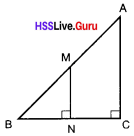(MN || AC)
∴ $$\frac{AM}{MB} = \frac{NC}{NB}$$
1 = $$\frac{NC}{NB}$$ ∴ CN = NB = 4
∴ MB = 5, NB = 4, MN = 3

Question 10.
In the figure ABC is a right angled triangle and M is the midpoint of AB. Prove that MN = V2AC and also prove that MN = MA = MB.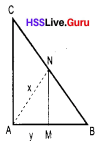MN = $$\sqrt{x^{2} – y^{2}}$$
AC = $$\sqrt{4x^{2} – 4y^{2}} = 2\sqrt {x^{2} – y^{2}}$$
AC = 2 × MN or MN = $$\frac{1}{2}$$AC
MC = $$\sqrt{x^{2} – 4^{2} + 4^{2}}$$; MC = X